intelliJ IDEA Java注释模板配置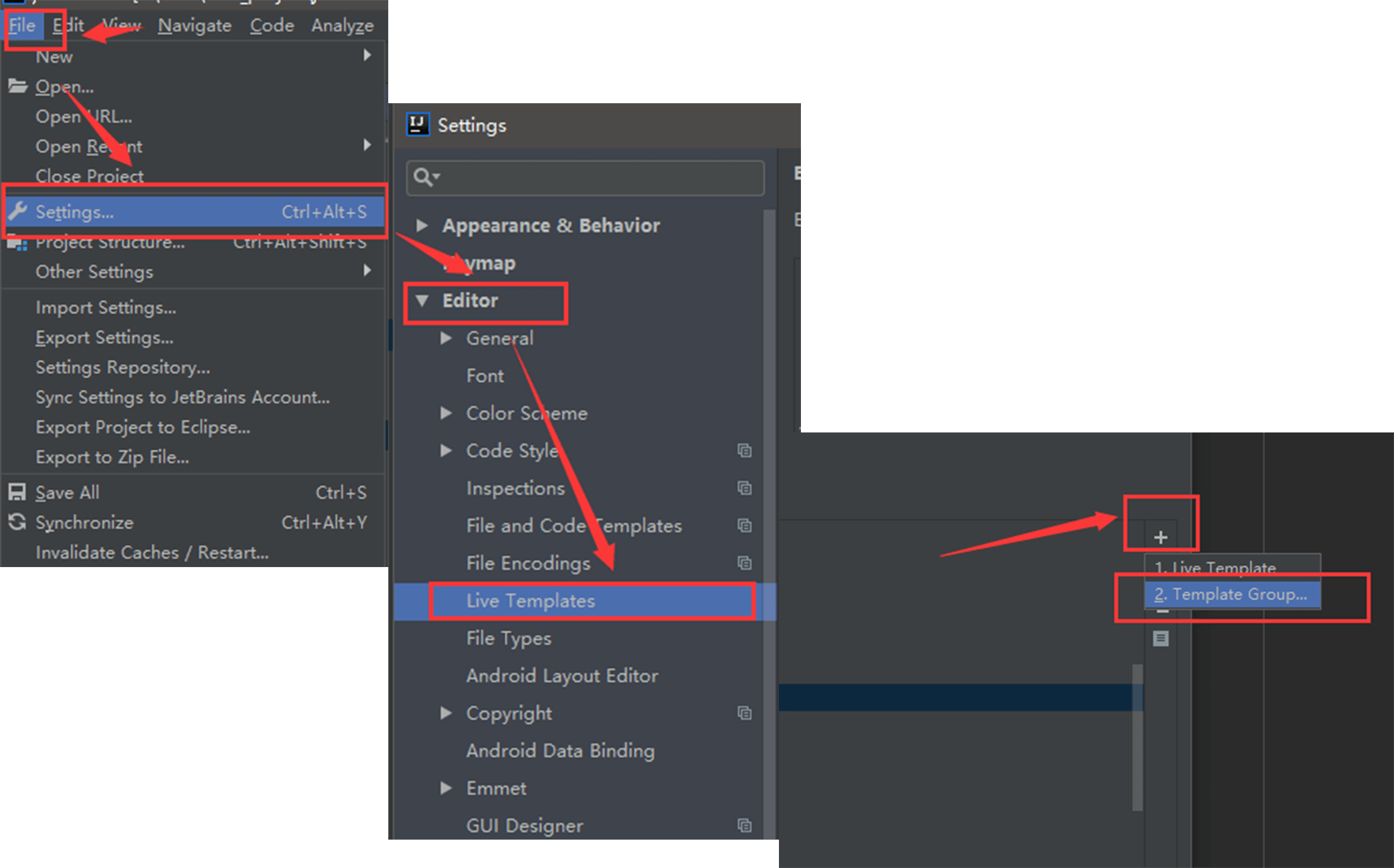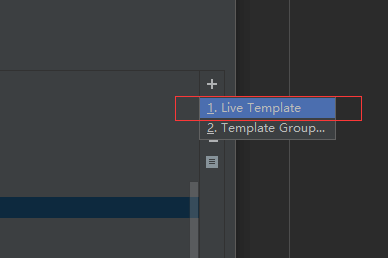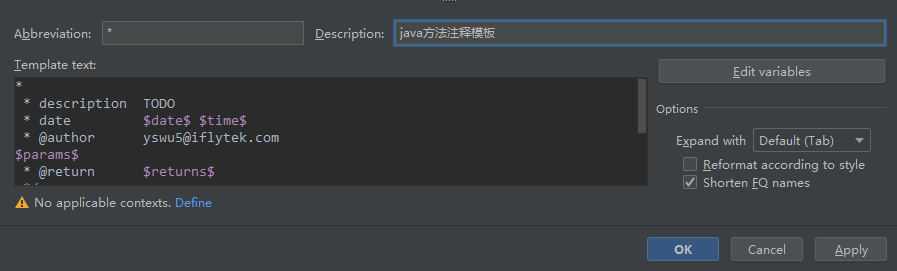Template text中需要我们输入模板内容，需要注意的是动态内容必须使用$包裹，否则无效。此处我设置的是方法的注释模板，内容如下： * * description TODO * date$datetime$* @author song@devsong.org$params$* @return$returns$*/ 由于我们针对的是Java的配置，所以输入模板内容后，点击左下角的Define设置可用的范围，直接勾选Java即可。如图: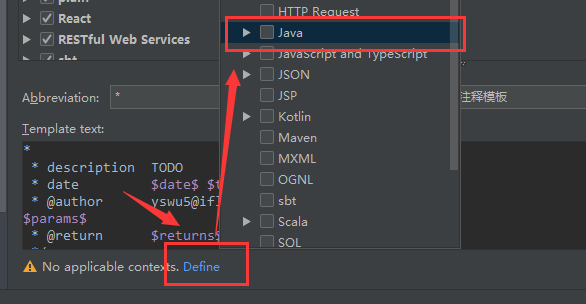然后点击Edit variables进行参数配置，配置如下：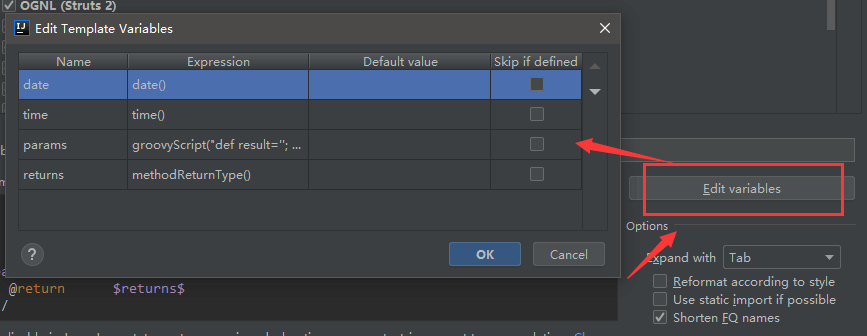params里面由于可能涉及多个参数，所以需要使用groovy脚本来处理,脚本内容如下： groovyScript("def result=''; def params=\"${_1}\".replaceAll('[\\\$|\\\$|\\\\s]', '').split(',').toList(); for(i = 0; i < params.size(); i++) {result+=' * @param       ' + params[i] + ((i < params.size() - 1) ? '\\n' : '')}; return result", methodParameters())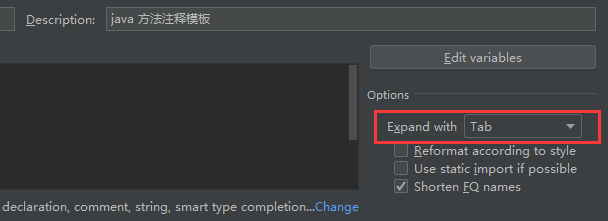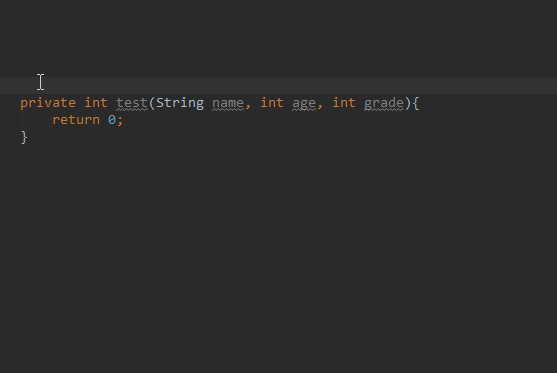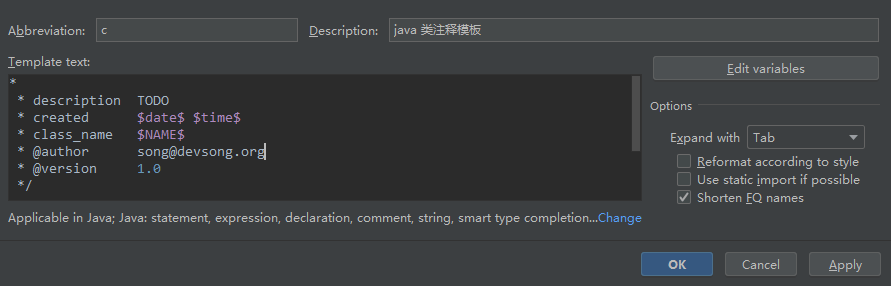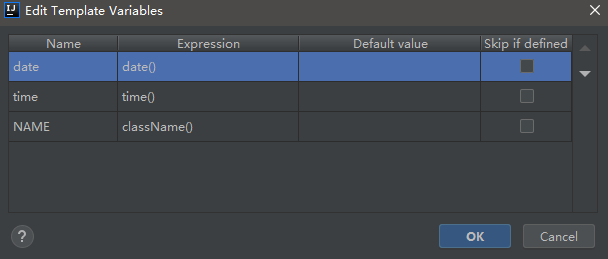*
* description  TODO
* created      $date$ $time$
* class_name   $NAME$
* @author      song@devsong.org
* @version     1.0
*/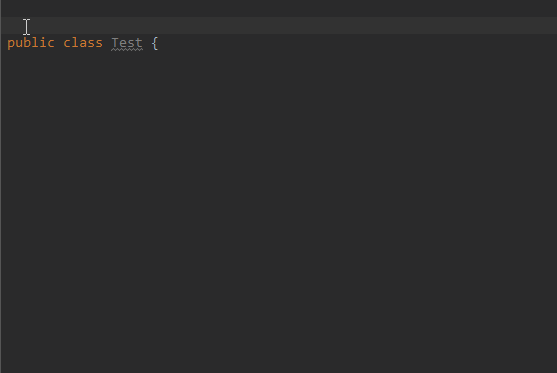### (1)方法注释模板 * * * $params$ * @return * @date $date$ $time$ * @author song@devsong.org */  ###params脚本 groovyScript("def result=''; def params=\"${_1}\".replaceAll('[\\\$|\\\$|\\\\s]', '').split(',').toList(); for(i = 0; i < params.size(); i++) {if(params[i] != ''){if(i == 0){result += '\\n'};result+=' * @param ' + params[i] + ' ' + (i+1) + ((i < params.size() - 1) ? '\\n' : '')}}; return result", methodParameters())  (2)类注释模板 * * * * @author song@devsong.org * @version 1.0 * @date$datetime$* @className$NAME\$ */ 

It's

*转载请注明出处，严禁非法转载。
https://www.devsong.org
QQ留言 邮箱留言